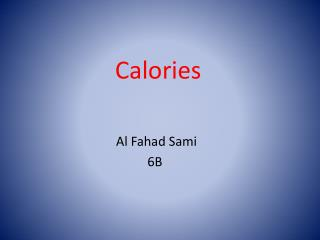DownloadDownload PresentationCalories

Calories

Télécharger la présentationCalories

- - - - - - - - - - - - - - - - - - - - - - - - - - - E N D - - - - - - - - - - - - - - - - - - - - - - - - - - -
Presentation Transcript

1. Calories Al Fahad Sami 6B

2. Calories Gained vs. Calories Burned

3. How many calories in a doughnut? There are over 200 calories in a glazed doughnut.

4. Chocolate doughnut Did you know that one chocolate doughnut has over 250 calories, and a glazed chocolate doughnut has 300 calories.

5. Number of calories burned depends on what speed your running at. In sports shops there are stuff that you put on your hand or legs to see how many calories you are burning while running.

6. The amount of calories that running burns per kilometer varies from person to person depending on factors such as : Weight , running speed , duration of running and whether you are a girl or a boy. The most important factor is weight

7. To find out how many calories burned when running Multiply your weight in kilograms by how many kilometers you ran and 1.036 Calories burned = weight (kg) × Km × 1.036

8. A person who weighs 52.3 kg and runs 1 km would burn : Calories burned = weight (kg) × km × 1.036 Calories burned = 52.3 × 1 × 1,036 = 54.1828 calories per 1 km

9. How far would that person have to run to burn the calories that are in a doughnut ? If he ate a glazed doughnut which has 200 calories , he needs to run for about 3.7 km . Calories = kg × km × 1.036 = 52.3 × ? × 1.036 = 200 First we multiply 52.3 × 1.036 = 54.1828 Then we divide 200 by 54.1828 200 ÷ 54.1828 = 3.69 km

10. Who do you think needs to run more to burn calories from a doughnut Let’s compare between two boys, the first boy’s weight is 40 kg while the second boy is 70kg Using the formula : weight × km × 1.036 First boy : 40 × 1 × 1.036 = 41.44 calories Then we divided 200 by 41.44 200 ÷ 41.44 = 4.8 km Second boy : 70 × 1 × 1.036 = 72.52 calories 200 divided by 72.52 200 ÷ 72.52 = 2.7 km

11. Have you ever wondered how many calories in French Fries !!! medium portion of French fries has 360 calories. So if you weight 52.3 kg and you want to know how many calories you burn when running Calories = weight (kg) × km × 1.036 = 52.3 × ? × 1.036 = 360 (52.3 × 1.036 = 54.1828 ) 54.1828 × ? = 360 so I can get the answer by dividing 360 by 54.1828 = 360 ÷ 54.1828 = 6.64 km So if you decided to eat medium French fries rather than a glazed doughnut you would have to run 2.95 kilometers more Km you run to burn French Fries subtract Km you run for doughnut 6.64 – 3.69 = 2.95 km

12. Knowing arithmetic with decimals help me make good decisions by : Calculating calories by adding up all the food that you have eaten in one day for an example. Trying to avoid food that have big calories, and even decimals add up together not only whole numbers Doing activities that burn more calories. Using the required formula to find out how many calories burned from each activity you do

13. Arithmetic with decimals help me develop these parts of the Learner Profile I become better thinker and knowledgeable. I think more about calories before I eat anything ; and I put in mind that even decimal calories add up together for all the food that I eat. I make better decisions in choosing food with low calories. I communicate with my friends and find out what activities burn more calories.

14. Sources I used : www.coolrunning.com www.runnersworld.com www.fitwatch.com www.caloriecount.com